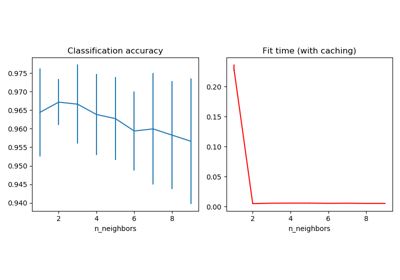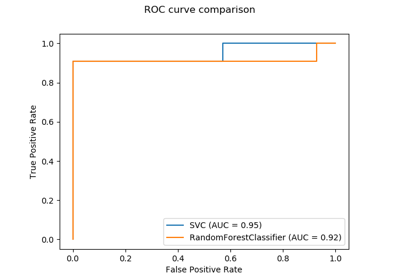# sklearn.neighbors.KNeighborsTransformer¶

class sklearn.neighbors.KNeighborsTransformer(mode='distance', n_neighbors=5, algorithm='auto', leaf_size=30, metric='minkowski', p=2, metric_params=None, n_jobs=1)[source]

Transform X into a (weighted) graph of k nearest neighbors

The transformed data is a sparse graph as returned by kneighbors_graph.

Read more in the User Guide.

New in version 0.22.

Parameters
mode{‘distance’, ‘connectivity’}, default=’distance’

Type of returned matrix: ‘connectivity’ will return the connectivity matrix with ones and zeros, and ‘distance’ will return the distances between neighbors according to the given metric.

n_neighborsint, default=5

Number of neighbors for each sample in the transformed sparse graph. For compatibility reasons, as each sample is considered as its own neighbor, one extra neighbor will be computed when mode == ‘distance’. In this case, the sparse graph contains (n_neighbors + 1) neighbors.

algorithm{‘auto’, ‘ball_tree’, ‘kd_tree’, ‘brute’}, default=’auto’

Algorithm used to compute the nearest neighbors:

Note: fitting on sparse input will override the setting of this parameter, using brute force.

leaf_sizeint, default=30

Leaf size passed to BallTree or KDTree. This can affect the speed of the construction and query, as well as the memory required to store the tree. The optimal value depends on the nature of the problem.

metricstring or callable, default=’minkowski’

metric to use for distance computation. Any metric from scikit-learn or scipy.spatial.distance can be used.

If metric is a callable function, it is called on each pair of instances (rows) and the resulting value recorded. The callable should take two arrays as input and return one value indicating the distance between them. This works for Scipy’s metrics, but is less efficient than passing the metric name as a string.

Distance matrices are not supported.

Valid values for metric are:

• from scikit-learn: [‘cityblock’, ‘cosine’, ‘euclidean’, ‘l1’, ‘l2’, ‘manhattan’]

• from scipy.spatial.distance: [‘braycurtis’, ‘canberra’, ‘chebyshev’, ‘correlation’, ‘dice’, ‘hamming’, ‘jaccard’, ‘kulsinski’, ‘mahalanobis’, ‘minkowski’, ‘rogerstanimoto’, ‘russellrao’, ‘seuclidean’, ‘sokalmichener’, ‘sokalsneath’, ‘sqeuclidean’, ‘yule’]

See the documentation for scipy.spatial.distance for details on these metrics.

pint, default=2

Parameter for the Minkowski metric from sklearn.metrics.pairwise.pairwise_distances. When p = 1, this is equivalent to using manhattan_distance (l1), and euclidean_distance (l2) for p = 2. For arbitrary p, minkowski_distance (l_p) is used.

metric_paramsdict, default=None

Additional keyword arguments for the metric function.

n_jobsint, default=1

The number of parallel jobs to run for neighbors search. If -1, then the number of jobs is set to the number of CPU cores.

Examples

>>> from sklearn.manifold import Isomap
>>> from sklearn.neighbors import KNeighborsTransformer
>>> from sklearn.pipeline import make_pipeline
>>> estimator = make_pipeline(
...     KNeighborsTransformer(n_neighbors=5, mode='distance'),
...     Isomap(neighbors_algorithm='precomputed'))


Methods

 fit(self, X[, y]) Fit the model using X as training data fit_transform(self, X[, y]) Fit to data, then transform it. get_params(self[, deep]) Get parameters for this estimator. kneighbors(self[, X, n_neighbors, …]) Finds the K-neighbors of a point. kneighbors_graph(self[, X, n_neighbors, mode]) Computes the (weighted) graph of k-Neighbors for points in X set_params(self, \*\*params) Set the parameters of this estimator. transform(self, X) Computes the (weighted) graph of Neighbors for points in X
__init__(self, mode='distance', n_neighbors=5, algorithm='auto', leaf_size=30, metric='minkowski', p=2, metric_params=None, n_jobs=1)[source]

Initialize self. See help(type(self)) for accurate signature.

fit(self, X, y=None)[source]

Fit the model using X as training data

Parameters
X{array-like, sparse matrix, BallTree, KDTree}

Training data. If array or matrix, shape [n_samples, n_features], or [n_samples, n_samples] if metric=’precomputed’.

fit_transform(self, X, y=None)[source]

Fit to data, then transform it.

Fits transformer to X and y with optional parameters fit_params and returns a transformed version of X.

Parameters
Xarray-like of shape (n_samples, n_features)

Training set.

yignored
Returns
XtCSR sparse graph of shape (n_samples, n_samples)

Xt[i, j] is assigned the weight of edge that connects i to j. Only the neighbors have an explicit value. The diagonal is always explicit.

get_params(self, deep=True)[source]

Get parameters for this estimator.

Parameters
deepbool, default=True

If True, will return the parameters for this estimator and contained subobjects that are estimators.

Returns
paramsmapping of string to any

Parameter names mapped to their values.

kneighbors(self, X=None, n_neighbors=None, return_distance=True)[source]

Finds the K-neighbors of a point. Returns indices of and distances to the neighbors of each point.

Parameters
Xarray-like, shape (n_queries, n_features), or (n_queries, n_indexed) if metric == ‘precomputed’

The query point or points. If not provided, neighbors of each indexed point are returned. In this case, the query point is not considered its own neighbor.

n_neighborsint

Number of neighbors to get (default is the value passed to the constructor).

return_distanceboolean, optional. Defaults to True.

If False, distances will not be returned

Returns
neigh_distarray, shape (n_queries, n_neighbors)

Array representing the lengths to points, only present if return_distance=True

neigh_indarray, shape (n_queries, n_neighbors)

Indices of the nearest points in the population matrix.

Examples

In the following example, we construct a NearestNeighbors class from an array representing our data set and ask who’s the closest point to [1,1,1]

>>> samples = [[0., 0., 0.], [0., .5, 0.], [1., 1., .5]]
>>> from sklearn.neighbors import NearestNeighbors
>>> neigh = NearestNeighbors(n_neighbors=1)
>>> neigh.fit(samples)
NearestNeighbors(n_neighbors=1)
>>> print(neigh.kneighbors([[1., 1., 1.]]))
(array([[0.5]]), array([]))


As you can see, it returns [[0.5]], and [], which means that the element is at distance 0.5 and is the third element of samples (indexes start at 0). You can also query for multiple points:

>>> X = [[0., 1., 0.], [1., 0., 1.]]
>>> neigh.kneighbors(X, return_distance=False)
array([,
]...)

kneighbors_graph(self, X=None, n_neighbors=None, mode='connectivity')[source]

Computes the (weighted) graph of k-Neighbors for points in X

Parameters
Xarray-like, shape (n_queries, n_features), or (n_queries, n_indexed) if metric == ‘precomputed’

The query point or points. If not provided, neighbors of each indexed point are returned. In this case, the query point is not considered its own neighbor.

n_neighborsint

Number of neighbors for each sample. (default is value passed to the constructor).

mode{‘connectivity’, ‘distance’}, optional

Type of returned matrix: ‘connectivity’ will return the connectivity matrix with ones and zeros, in ‘distance’ the edges are Euclidean distance between points.

Returns
Asparse graph in CSR format, shape = [n_queries, n_samples_fit]

n_samples_fit is the number of samples in the fitted data A[i, j] is assigned the weight of edge that connects i to j.

Examples

>>> X = [, , ]
>>> from sklearn.neighbors import NearestNeighbors
>>> neigh = NearestNeighbors(n_neighbors=2)
>>> neigh.fit(X)
NearestNeighbors(n_neighbors=2)
>>> A = neigh.kneighbors_graph(X)
>>> A.toarray()
array([[1., 0., 1.],
[0., 1., 1.],
[1., 0., 1.]])

set_params(self, **params)[source]

Set the parameters of this estimator.

The method works on simple estimators as well as on nested objects (such as pipelines). The latter have parameters of the form <component>__<parameter> so that it’s possible to update each component of a nested object.

Parameters
**paramsdict

Estimator parameters.

Returns
selfobject

Estimator instance.

transform(self, X)[source]

Computes the (weighted) graph of Neighbors for points in X

Parameters
Xarray-like of shape (n_samples_transform, n_features)

Sample data

Returns
XtCSR sparse graph of shape (n_samples_transform, n_samples_fit)

Xt[i, j] is assigned the weight of edge that connects i to j. Only the neighbors have an explicit value. The diagonal is always explicit.

## Examples using sklearn.neighbors.KNeighborsTransformer¶